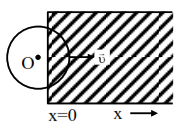# A constant magnetic fieldQuestion:

A constant magnetic field of $1 \mathrm{~T}$ is applied in the $x>0$ region. A metallic circular ring of radius $1 \mathrm{~m}$ is moving with a constant velocity of $1 \mathrm{~m} / \mathrm{s}$ along the $\mathrm{x}$-axis. At $\mathrm{t}=0 \mathrm{~s}$, the centre of $\mathrm{O}$ of the ring is at $\mathrm{x}=-1 \mathrm{~m} .$ What will be the value of the induced emf in the ring at $\mathrm{t}=1 \mathrm{~s}$ ? (Assume the velocity of the ring does not change.)1. $1 \mathrm{~V}$

2. $2 \pi V$

3. $2 \mathrm{~V}$

4. $0 \mathrm{~V}$

Correct Option: , 3

Solution:

$e m f=B L V$

$=1 \cdot(2 \mathrm{R}) \cdot 1$

$=2 \mathrm{~V}$